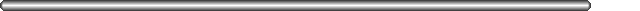# CLASS 13## Placing variables on the stack

We should always refer to variables in terms of the frame pointer, since it won't change during the execution of the subroutine. The stack pointer might be modified during the execution of the subroutine.

Say we want to place the following variables on the stack:

```	int a, b;
char ch;
short c;
int d;
```
How would the stack look and what are the offsets? Remember we address words, etc, by the lowest numbered byte contained:
```	fp - 16: |   |   |   |   | (d)
fp - 12: |   |   | (c)
fp - 10: | X |
fp - 9:  |   | (ch)
fp - 8:  |   |   |   |   | (b)
fp - 4:  |   |   |   |   | (a)
0   1   2   3
```
We need the byte location fp - 10 to align the rest of the stack, halfword on mod 2 boundaries, word on mod 4, etc.

Another Example: Suppose we have the following C declarations:

```int a, b, c ;
char d;
short e;
char f;
int g;
```

The notation for the variables on the stack is _s. e.g. a_s, b_s, c_s... and so on.

Corresponding to the C declarations, the offsets on the stack will be defined as follows:

```define(a_s, -4)
define(b_s, -8)
define(c_s, -12)
define(d_s, -13)
define(e_s, -16)
define(f_s, -17)
define(g_s, -24)
```

Now, the initial declaration will be:

```save %sp, ((-64 - 24) & -8), %sp
==> save %sp, -88 & -8 , %sp
==> save %sp, -88 , %sp
```
And the variables can be loaded into the registers as:
```ld   [%fp + a_s], %o0
ldsh [%fp + e_s], %o1
ldsb [%fp + d_s], %o2
and so on.......
```

Another Example:

```int a, b;
char c;
int d, e ;
register int x, y, z;
x = 17;
y = -5;
```
The sparc code for these definitions is as follows:
```define(a_s, -4)
define(b_s, -8)
define(c_s, -9)
define(d_s, -16)
define(e_s, -20)
define(x_r, l0)
define(y_r, l1)
define(z_r, l2)
```
Hence, the starting instruction of main will be:
```save %sp, ((-64 - 20) & -8), %sp
==> save %sp, (-84 & -8), %sp
==> save %sp, -88, %sp

mov 17, x_r
mov -5, y_r
```

Now, if we want to increment the value of a by 1, we have to type the following commands,

```ld [%fp + a_s], %o0
inc %o0
st %o0, [%fp + a_s]
```
What the previous example shows is that it is not possible to operate on values directly in memory, and we first have to get them from memory into a register. Only then can we operate on them. Also, at the end of the operation, the values have to be stored back into memory, or else the next instruction might be reading a wrong value from that memory location. This is called maintaining "memory consistency".

## M4 macros (or, how to read the examples in the book)

It is tedious making sure that data is aligned. So the book uses m4 macros to calculate offsets.

There are m4 macros that name stack variables, place them on the stack, and make sure they are properly aligned. They are:

```	local_var  (This goes at the beginning of the program or subr)
var(name, size)  (This sets aside aligned space on the stack)
```
The book starts a program with:
```	begin_main
```
which translates to
```	.global main
main:
save %sp, (-64 + <stack space needed>) & -8, %sp
```
We also have the macro
```	end_main

```
which does the
``````mov 1, %g1
ta 0```
```
needed at the end of a main program.

NOTE : WE WILL NOT BE MAKING USE OF THE MACRO NOTATIONS FOR THE PURPOSE OF THIS COURSE

## Example of Stack use

Below, there is another example of stack use. Here, we specify the locations of the variables sh and i using the constant assignments, instead of the macro declarations used in the text.
```     1	main()
2	{
3	        short int sh;
4	        register int i;
5
6	        i = 1;
7		sh = 0;
8
9	        while (sh<100)
10	        {
11	                i = i + i;
12			sh++;
13	        }
14	}
```
This translates to:
```	!local variables

/* line 3 */
sh_s = -2

/* line 4 */
! l0 = %l0

.global	_main
_main:	save	%sp, -72, %sp

/* line 6 */
mov 	1, %l0

/* line 7 */
clr	%o0
sth	%o0, [%fp + sh_s]

/* line 9; while loop */
ldsh	[%fp + sh_s], %o1
ba 	test
cmp	%o1, 100

loop:
sth	%o1, [%fp + sh_s]
cmp	%o1, 100

/* bottom of while loop */
test:
bl,a	loop
nop

/* line 14 */
mov	1, %g1
ta	 0
```